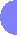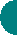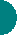HomePublicationsOR ResearchCurriculum Vitae# Research in Operations Research- Reuven Chen

## TAU_P_CENTER - Optimal Solutions for the P-Center Problem

Improved Relaxation - Set Covering Subproblem

CPLEX_tau_p_center_discrete - This C code can be used in order to optimally solve the discrete version of the P-center problem, where the set of demand points and the set of potential service points are the same.

CPLEX_tau_p_center_discrete_euc - This C code can be used in order to optimally solve the discrete version of the P-center problem, where the distances between the demand points and the potential service points are Euclidean. We also assume that the set of demand points and the set of potential service points are the same.

CPLEX_tau_p_center_cont_euc - This C code can be used in order to optimally solve the continuous version of the P-center problem, where the distances are Euclidean.

Improved Relaxation - Ilhan et al.'s Feasibility Subproblem

Reverse Relaxation - Set Covering Subproblem

Reverse Relaxation - Feasibility Subproblem

Binary Relaxation - Set Covering Subproblem

Binary Relaxation - Feasibility Subproblem